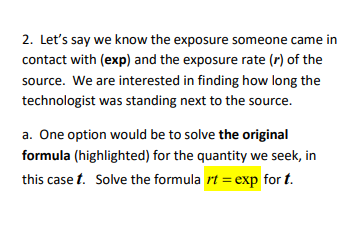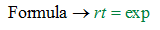# 2. Let's say we know the exposure someone came incontact with (exp) and the exposure rate (r) of thesource. We are interested in finding how long thetechnologist was standing next to the source.a. One option would be to solve the originalformula (highlighted) for the quantity we seek, inthis case t. Solve the formula rt = exp for t.

Question
2 views

Im so confused on this, I really don't know where to go from here. I got t= exp/r for my equation, but what do i do from there??? Please help !help_outlineImage Transcriptionclose2. Let's say we know the exposure someone came in contact with (exp) and the exposure rate (r) of the source. We are interested in finding how long the technologist was standing next to the source. a. One option would be to solve the original formula (highlighted) for the quantity we seek, in this case t. Solve the formula rt = exp for t. fullscreen
check_circle

Step 1

Given, exposure (exp) and the exposure rate (r)...

### Want to see the full answer?

See Solution

#### Want to see this answer and more?

Solutions are written by subject experts who are available 24/7. Questions are typically answered within 1 hour.*

See Solution
*Response times may vary by subject and question.
Tagged in

### Calculus